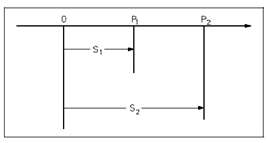## Differentials and Derivatives Assignment Help

Assignment Help: >> Calculus - Differentials and Derivatives

Differentials and Derivatives:

One of the most general encountered applications of the mathematics of dynamic systems involves the relationship among position and time for a moving object.  Below figure represents an object moving in a straight line from position P1 to position P2.  The distance to P1 from a fixed reference point, point 0, along with the line of travel is represented through S1; the distance to P2 from point 0 by S2.Figure: Motions Between Two Points

If the time recorded by a clock, while the object is at position P1  is t1, and if the time when the object is at position P2  is t2, then the average velocity of the object among points P1  and P2 equals the distance traveled, divided by the elapsed time.

Vav = S2 - S1/t2 -t1                                         . . .(1)

If positions P1 and P2 are close together, the distance traveled and the elapsed time is little. The symbol Δ, the Greek letter delta, is used to indicate changes in quantities.  Therefore, the average velocity while positions P1 and P2 are close together is often written using deltas.

Vav = ΔS/Δt = S2 - S1/t2 -t1                       . . .(2)

While the average velocity is frequent an significant quantity, in several cases it is essential to know the velocity at a given instant of time.  This velocity, called the instantaneous velocity, is not the similar as the average velocity, unless the velocity is not changing along with time.

 Combined expression Displacement Versus Time Example